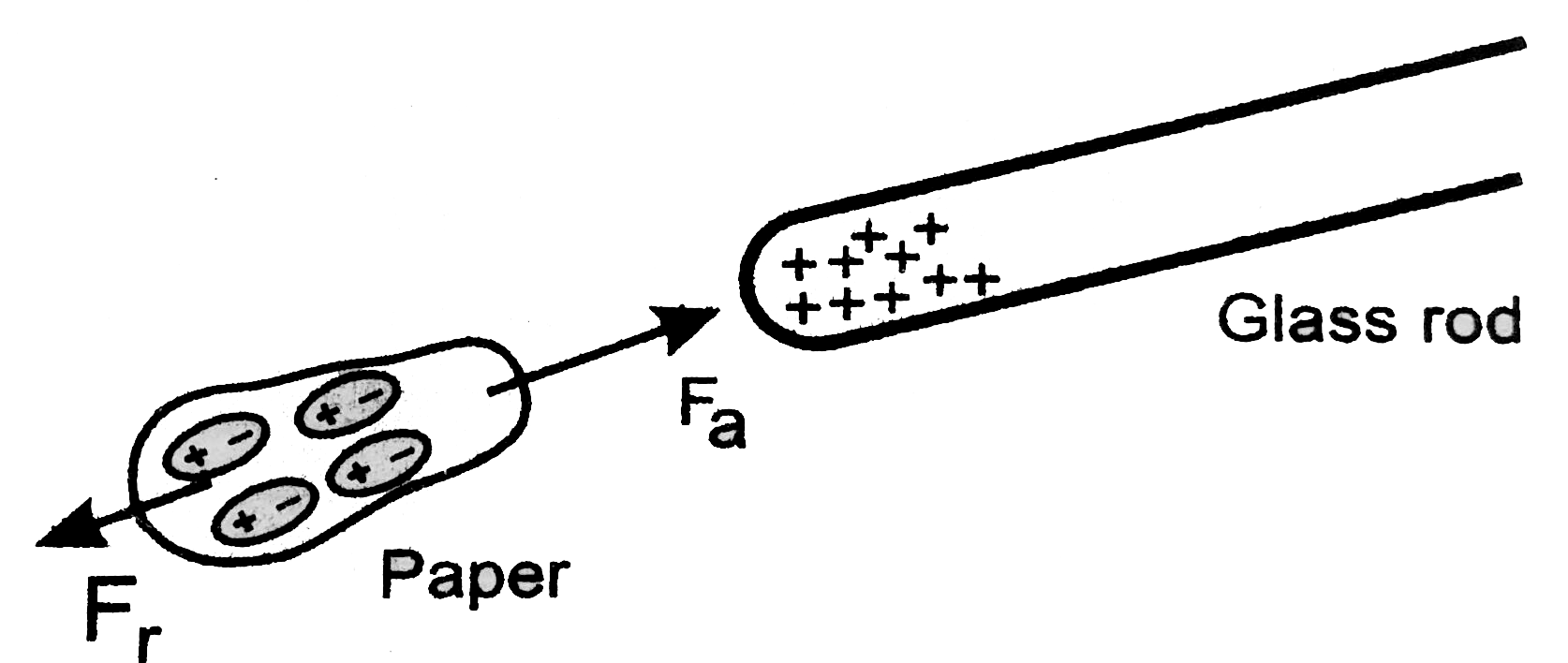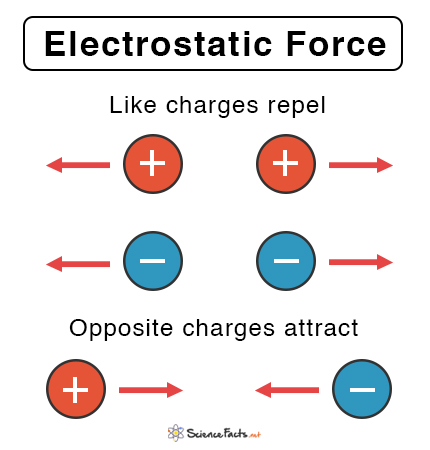# Electric Charge And Electric Force Study Guide

INTRODUCTION

It’s always entertaining to see fundamental science in action. Observing pieces of paper being drawn to a rubbed glass rod, for example, is always fascinating. But have you ever wondered why this occurs? What is it about the glass rod that attracts the paper shards? Answers to these questions can be found at the atomic level. These bodies are attracted to each other due to the presence of opposing charges in them. Let’s take a closer look at this subject.Source

## WHAT IS AN ELECTRIC CHARGE?

• The electric charge definition states that it is a property of atomic particles that allows them to experience a force when placed in an electric or a magnetic field.

• There are two types of electric charges.

1. The charge that is positive (+)
2. The charge that is negative (-)
• A negative charge indicates that an item has more electrons than protons, whereas a positive charge indicates that an object has more protons than electrons.

• These electric charges are usually transported by charge carriers, protons and electrons.

• These charges also have their own distinct properties.

• The following are some examples of charge properties:
2. Electric charge conservation
3. Electric charge quantification

## WHAT IS ELECTRICAL FORCE?

• The electric force definition states that it is the repulsive or attractive relationship between any two charged things.
• Newton’s equations of motion define the impact and effects of any force on a particular body, just as they do for any other force.
• This means that the electric force is determined by the electric charge rather than the mass of the item.
• This also means that the electric force between two protons or two electrons at an equal distance will always be equal.Source

## RELATION BETWEEN ELECTRICAL FORCE AND ELECTRICAL CHARGE

• Coulomb’s law is an experimental rule that determines the amount of force among two electrically charged particles that are static.
• The electrostatic force, also known as Coulomb’s force, is the electric force between two stationary charged bodies.
• The quantity of electrostatic force among stationary charges is described by Coulomb’s law.
• The electrostatic interaction force is inversely proportional to the square distance between the two charges and directly proportional to the point charges themselves.

## THE FORMULA FOR ELECTRICAL FORCE

The formula for electrical force is:

F= K (q1. q2) / r2

Where,

• F is the electrical force,
• q1 and q2 are the amounts of charge,
• And r is the distance between two charged bodies.
• The current electricity that powers all the present technologies is caused due to electric force.

# SUMMARY

• Electric charge is the feature of subatomic particles that enables them to experience a force when put in an electric and magnetic field.
• The electric force is the repulsive or attractive relationship between any two charged things.
• The formula of electrical force is

F= K (q1. q2) / r2

## FAQs

Q. What is the relationship between electric charge and force?

The electrical force between two charged bodies is directly proportional to the charge carried by the bodies.

Q. What is the electric force?

The electric force is the repulsive or attractive relationship between any two charged things.

We hope you enjoyed studying this lesson and learned something cool about Electric Charge And Electric Force! Join our Discord community to get any questions you may have answered and to engage with other students just like you! We promise, it makes studying much more fun!😎

## REFERENCE

1. Electric Charge and Electric Force: https://www.ck12.org/physics/electric-charge-and-electric-force/lesson/Forces-on-Charged-Objects-PHYS/?referrer=concept_details Accessed 18th April 2022.
2. Electrostatics: https://en.wikipedia.org/wiki/Electrostatics Accessed 18th April 2022.
]]>